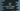# How to find the product of digits of a number in Java## How to find the product of digits of a number in Java:

We can iterate over the digits of a number and multiply all of the digits to find the final product. The algorithm works in a similar way in all programming languages. If you know how to write the program in any other language, it won’t be difficult to write it in Java.

I will explain how the algorithm works and I will also show you how to write the Java program in this post.

### Algorithm to find the product of the digits of a number:

We can use the below algorithm to find the product of digits of a given number:

1. Take the number as input from the user.
2. Create a copy of the number. The algorithm will work on the copied number.
3. Run one loop until the number becomes 0.
4. Initialize one variable as 1 to hold the final product of the digits of the number.
5. Inside the loop,
1. Get the rightmost digit of the number by using the modulo operator.
2. Multiply the digit by the product variable and assign that value to the product variable.
3. Remove the rightmost digit from the number.
6. Once the loop will end, the product variable will hold the final product of the digits of the number.

### Example 1: Java program to find the product of digits of a number:

The following Java program uses the above algorithm to find the product of digits of a user-input number:

``````import java.util.Scanner;

public class Main {

public static void main(String[] args) {
Scanner scanner = new Scanner(System.in);
int num, copyNum, lastDigit, product = 1;

System.out.println("Enter a number: ");
num = scanner.nextInt();

copyNum = num;

while (copyNum > 0) {
lastDigit = copyNum % 10;
product *= lastDigit;
copyNum /= 10;
}

System.out.println("Product of all digits of " + num + " is: " + product);
}
}``````

In this program,

• We created one object of Scanner class to read the user input values.
• The num variable holds the user input number, the copyNum variable is used to hold the copy of the number, the lastDigit variable is used to hold the last digit of the number and the product variable is used to hold the product of all digits of the number. The product variable is initialized as 1.
• In the while loop, it finds the last digit of the number and multiplies it with the product variable. It removes the last digit of the *copyNum variable on each iteration.
• At the end of the program, it prints the result.

If you run this program, it will give results as below:

``````Enter a number:
55555
Product of all digits of 55555 is: 3125``````

### Example 2: Java program to find the product of digits of a number by using a for loop:

We can also use a for loop to find the product of the digits of a number. This works almost similar to the above program.

``````import java.util.Scanner;

public class Main {

public static void main(String[] args) {
Scanner scanner = new Scanner(System.in);
int num, copyNum, lastDigit, product = 1;

System.out.println("Enter a number: ");
num = scanner.nextInt();

copyNum = num;

for (; copyNum > 0; copyNum /= 10) {
lastDigit = copyNum % 10;
product *= lastDigit;
}

System.out.println("Product of all digits of " + num + " is: " + product);
}
}``````

The initialization part of the for loop is empty as we are not initializing anything. It will run until the value of copyNum is greater than 0 and at the end of each iteration, it removes the last digit of the number.

You will get similar results if you run this program.